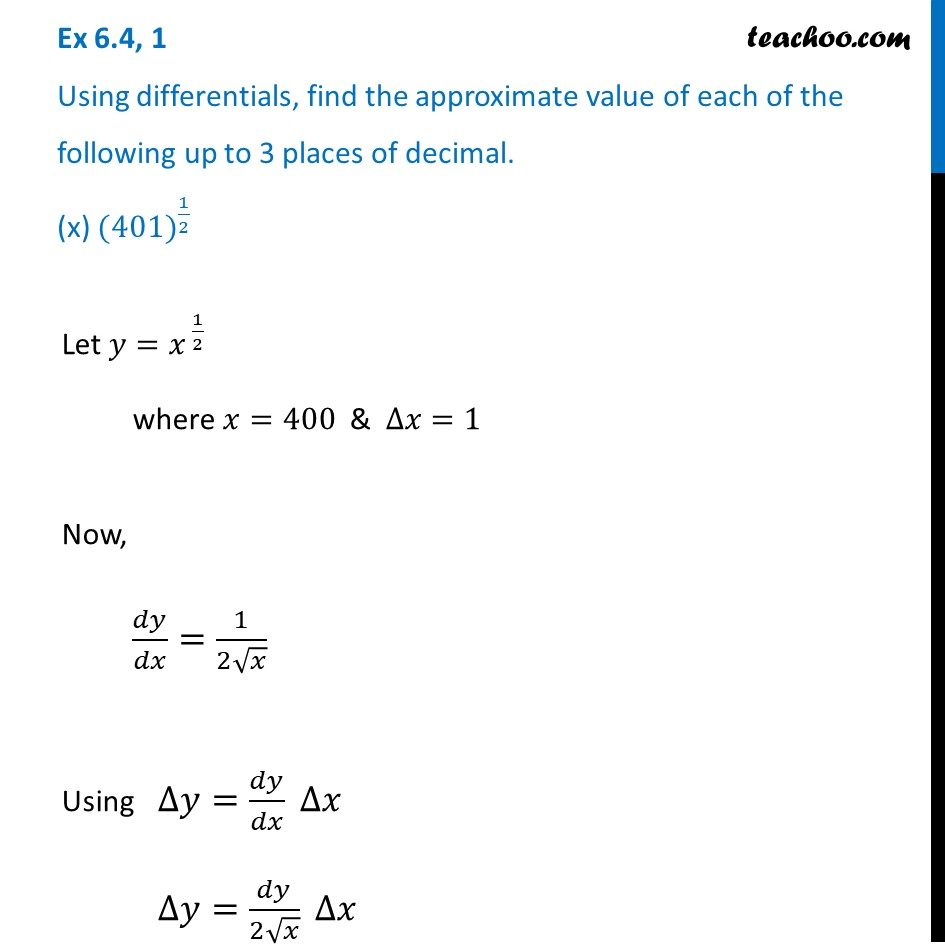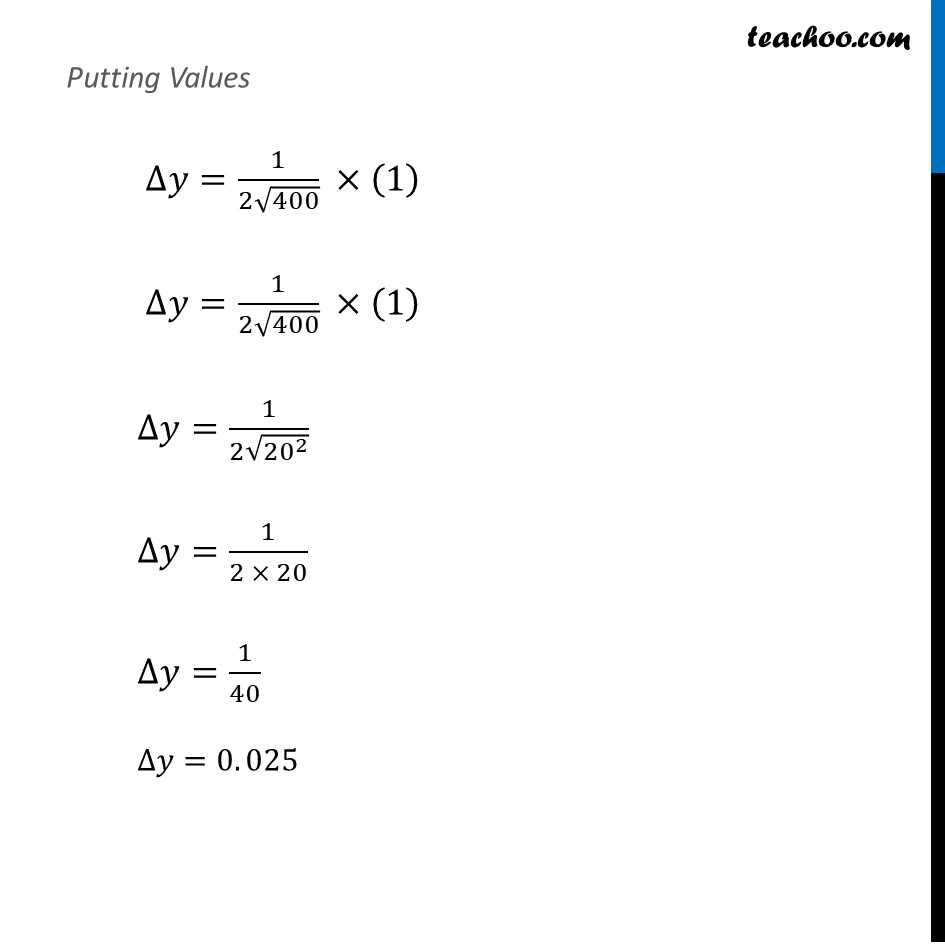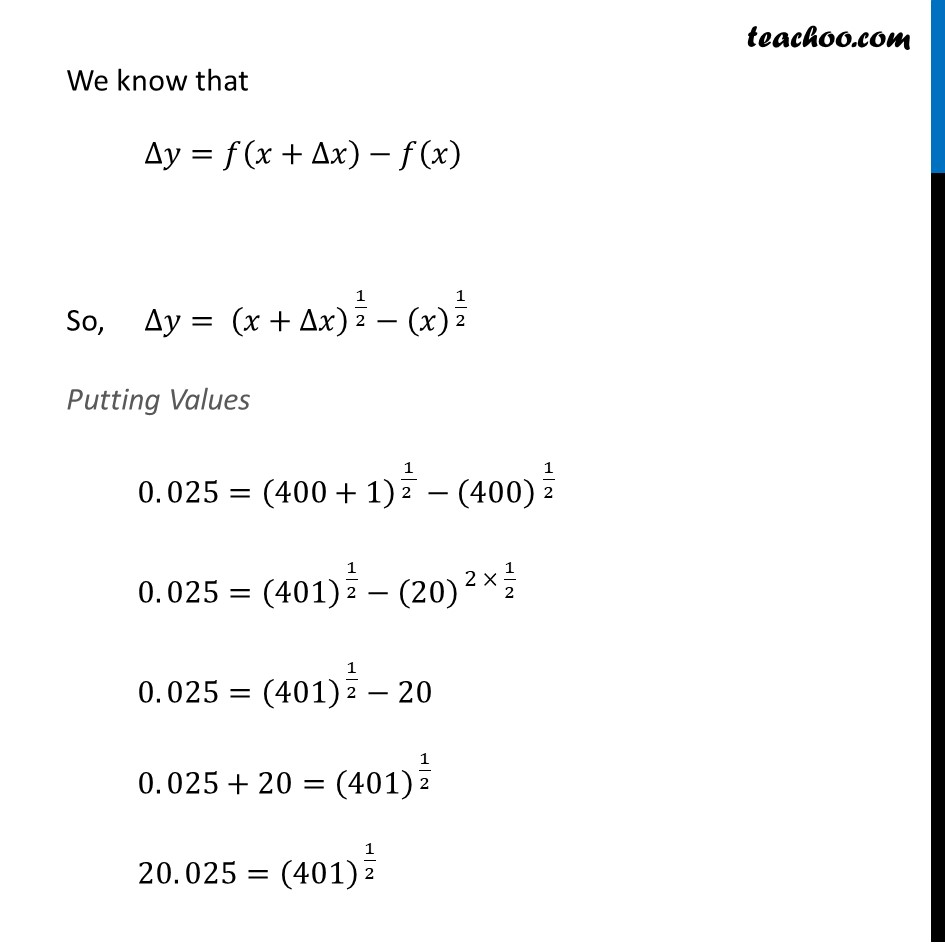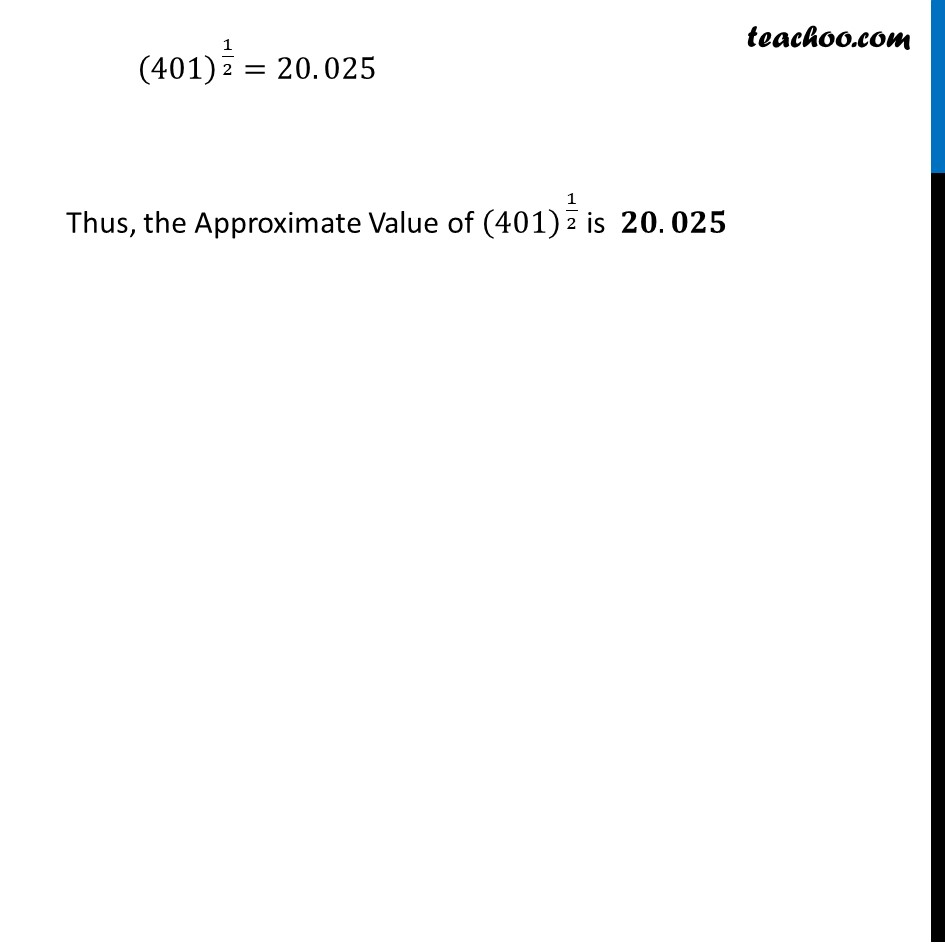Approximations (using Differentiation)

Chapter 6 Class 12 Application of Derivatives
Serial order wiseLearn in your speed, with individual attention - Teachoo Maths 1-on-1 Class

### Transcript

Question 1 Using differentials, find the approximate value of each of the following up to 3 places of decimal. (x) 〖(401)〗^(1/2)Let 𝑦=𝑥^( 1/2) where 𝑥=400 & ∆𝑥=1 Now, 𝑑𝑦/𝑑𝑥=1/(2√𝑥) Using ∆𝑦=𝑑𝑦/𝑑𝑥 ∆𝑥 ∆𝑦=𝑑𝑦/(2√𝑥) ∆𝑥 Putting Values ∆𝑦=1/(2√400) ×(1) ∆𝑦=1/(2√400) ×(1) ∆𝑦=1/(2√(20^2 )) ∆𝑦=1/(2 × 20) ∆𝑦=1/40 ∆𝑦=0. 025 We know that ∆𝑦=𝑓(𝑥+∆𝑥)−𝑓(𝑥) So, ∆𝑦= (𝑥+∆𝑥)^( 1/2)−(𝑥)^( 1/2) Putting Values 0. 025=(400+1)^( 1/(2 ))−〖(400) 〗^(1/2) 0. 025=(401)^( 1/2)−(20)^( 2 × 1/2) 0. 025=(401)^( 1/2)−20 0. 025+20=(401)^( 1/2) 20. 025=(401)^( 1/2) We know that ∆𝑦=𝑓(𝑥+∆𝑥)−𝑓(𝑥) So, ∆𝑦= (𝑥+∆𝑥)^( 1/2)−(𝑥)^( 1/2) Putting Values 0. 025=(400+1)^( 1/(2 ))−〖(400) 〗^(1/2) 0. 025=(401)^( 1/2)−(20)^( 2 × 1/2) 0. 025=(401)^( 1/2)−20 0. 025+20=(401)^( 1/2) 20. 025=(401)^( 1/2)